# Donut Delight

## Sparking Curiosity to Fuel Sense Making of Multiplication and Division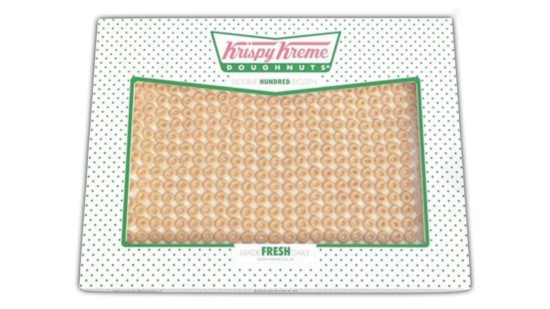When one of our district math leads, Brennan Jones asked me to brainstorm some ways we could help his staff engage in some professional development around division and incorporate a 3 act math task into the learning, I immediately thought of some contexts where arrays, base ten blocks and area models could be used to help attack this concept. I was thinking about a box of Coca-Cola, jars of peppers and many other ideas prior to settling on the idea of donuts in a box. It was then that I remembered that Graham Fletcher, Mike Wiernicki, YummyMath and others had explored the Krispy Kreme Double Hundred Dozen box of doughnuts in the past (read about it here). However, these innovative mathletes had approached the problem from the angle of multiplication and possibly some extension questions related to proportional reasoning. Brennan and I thought that we might be able to take that idea and make some connections to division.

This 3 act math task was designed with the idea of accessing student prior knowledge of multiplication and then connecting that knowledge to division including the use of open area models, repeated subtraction and then connecting these to a flexible division algorithm that is considered to be a more accessible algorithm for use by students with varying abilities.

## Curriculum Connections:

### Primary

Kindergarten, grade 1 and grade 2 are not explicitly covered in this task, however it would be reasonable to modify this task to make useful for these levels as well.

• Grade 3 – NS1 – divide whole objects and sets of objects into equal parts, and identify the parts using fractional names (e.g., one half; three thirds; two fourths or two quarters), without using numbers in standard fractional notation;
• Grade 3 – NS3 – relate multiplication of one-digit numbers and division by one-digit divisors to real-life situations, using a variety of tools and strategies (e.g., place objects in equal groups, use arrays, write repeated addition or subtraction sentences) (Sample problem: Give a real-life example of when you might need to know that 3 groups of 2 is 3 x 2.);

### Junior

• Grade 4 – NS3 – multiply two-digit whole numbers by one-digit whole numbers, using a variety of tools (e.g., base ten materials or drawings of them, arrays), student-generated algorithms, and standard algorithms;
• Grade 4 – NS3 – divide two-digit whole numbers by one-digit whole numbers, using a variety of tools (e.g., concrete materials, drawings) and student-generated algorithms;
• Grade 5 – NS3 – multiply two-digit whole numbers by two-digit whole numbers, using estimation, student-generated algorithms, and standard algorithms;
• Grade 6 – NS1 – solve problems involving the multiplication and division of whole numbers (four-digit by two-digit), using a variety of tools (e.g., concrete materials, drawings, calculators) and strategies (e.g., estimation, algorithms);

### Intermediate

• Grade 7 – NS3 – demonstrate an understanding of rate as a comparison, or ratio, of two measurements with different units (e.g., speed is a rate that compares distance to time and that can be expressed as kilometres per hour);
• Grade 8 – NS3 – identify and describe real-life situations involving two quantities that are directly proportional (e.g., the number of servings and the quantities in a recipe, mass and volume of a substance, circumference and diameter of a circle);
• Grade 9 Applied – NA1 – solve for the unknown value in a proportion, using a variety of methods (e.g., concrete materials, algebraic reasoning, equivalent ratios, constant of proportionality);
• Grade 9 Academic – NA2 – solve problems requiring the manipulation of expressions arising from applications of percent, ratio, rate, and proportion;;

## Act 1: Sparking Curiosity – Introduce the Task

Consider showing students the act 1 video from an episode of Fast Food Mania. The original, longer version is here.

Once the video is complete, show students this image if they are in an Ontario grade 5 classroom and ready for multiplying two-digit by two-digit numbers using a variety of strategies: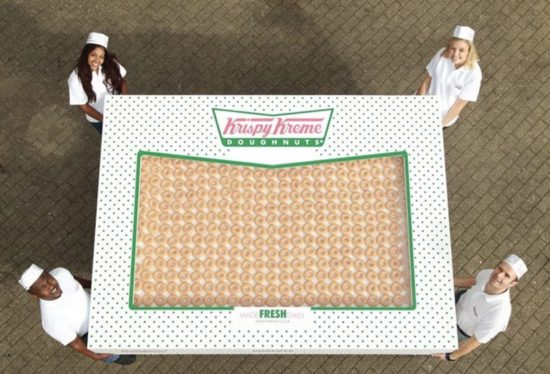If students are in an Ontario grade 4 classroom or lower, you might consider using this image: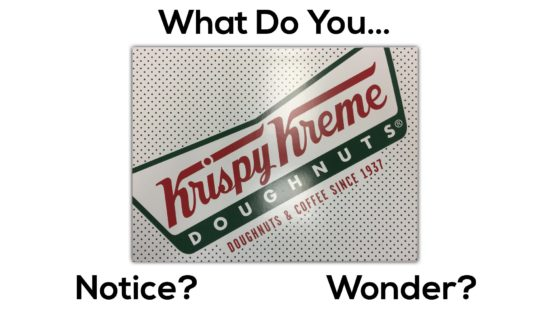Then ask students to do a rapid write of what they notice and what they wonder.

Students will then share out their noticings and wonderings while I jot their ideas down on the whiteboard.

Some noticings and wonderings that have come up when I’ve used the task with the huge box of donuts include:

• How many donuts are in that box?
• How heavy is the box?
• Those people look really small.
• Is this a real picture?
• How many calories are in that box?

For those who wish to go there, you can check out this article that covers the event at which this donut box was prepared for.

While we may explore some other wonderings, the first question I intend to address is:

How many donuts are in that box?

With manipulatives and/or paper/whiteboards already out on their tables, I would then give students some time to make a prediction and discuss with their neighbours and/or group.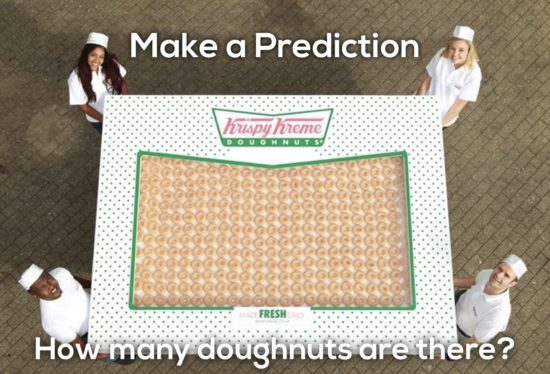If you’re using the small box of donuts with your class, you might show this image for predictions instead.

## Act 2: Reveal Some Information

After students have shared out their predictions, I would show them some information, depending on the group I’m working with.

### Version 1: Friendly Numbers Dimensions

If I want to use friendly numbers for students who are just beginning to multiply two-digit by two-digit numbers, I might use this image:### Version 2: Actual Dimensions (Less Friendly)

If students are ready for more of a challenge (and the actual dimensions of this box of Krispy Kreme doughnuts), I might use this image:

### Version 3: Smaller Box of Donuts

If students are in an Ontario grade 4 classroom or lower, you might give them a look at these images:

With these dimensions, you will offer students an opportunity to utilize multiplication strategies that might differ from student to student. Depending on where students are relative to concreteness fading, they may choose to concrete materials like base ten blocks; a visual representation such as drawing base ten blocks, an area model, or Japanese multiplication; or a symbolic representation such as partial products or the standard algorithm.

## Act 3: The Big Reveal

### Version 1: Reveal Friendly Number Solution

After students share out their strategies (see some exemplar strategies in “version 2” below), it is likely they will have arrived at a total of 600 donuts: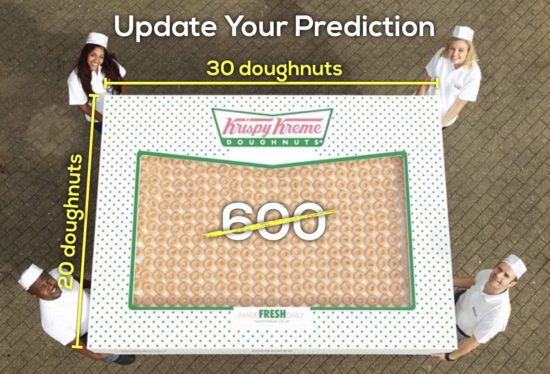Students may look confused wondering how the answer could not be 600. As they discuss with their groups, I’ll proceed to show them this image which shows a zoomed in photo of the box that states: “DOUBLE HUNDRED DOZEN”.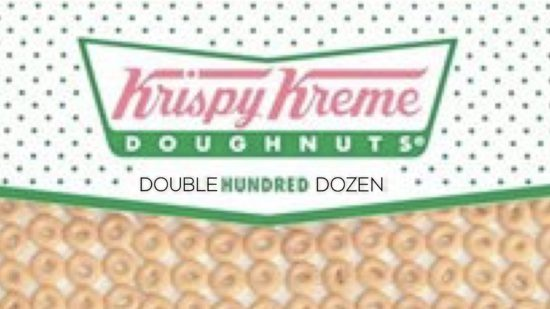Then, I let kids discuss and decide if they want to take some time to update their answers.

Hopefully, after consulting with their peers, they will arrive at an answer of 2,400 donuts.

### Version 2: Reveal Actual Dimension Solution

If you used the actual dimensions, students should arrive at a final answer of 800 donuts.

## Fuelling Sense Making

One of the most important realizations I’ve made recently is the importance of maximizing the opportunity to help students make sense of the math we are exposing them to. We do so much work to spark curiosity in order to engage our students in our lesson, we can’t miss the opportunity to help them visualize different mathematical models that exist within one seemingly simple mathematical scenario.

Here are some possible tools and representations I’ve observed and I now model when I’ve used this task with students and teachers. Hopefully, you see some of them in your classroom. If not, what a great opportunity to expose students to new strategies and representations to broaden their mathematical perspective.

#### Multiplication Strategy #1 – Using 100 Flats as 4 Groups of 25

In this multiplication strategy, some students make the connection that there are 4 groups of 25 in 100. Therefore, with the base ten blocks on the table, they can quickly use skip counting or other multiplicative thinking strategies to determine how many groups of 100 are in 32 groups of 25.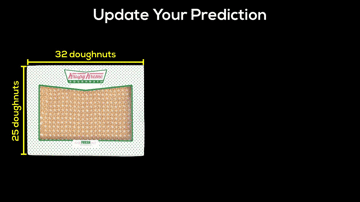#### Multiplication Strategy #2 – Multiplication Array With Base 10 Blocks

In this multiplication strategy, students create a multiplication array consisting of rows and columns with the factors 25 and 32. This is a great strategy that progresses nicely to the standard algorithm for multiplication.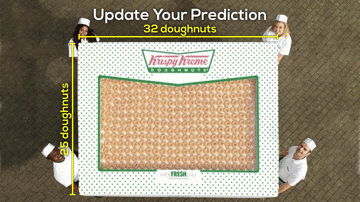#### Multiplication Strategy #3 – Connecting Base 10 Blocks to Japanese Multiplication

In this multiplication strategy, a connection can be made from a multiplication array using concrete manipulatives like base ten blocks to a visual representation, such as Japanese multiplication.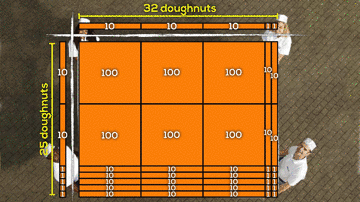Follow this link to watch a full video explanation of Japanese multiplication.

#### Multiplication Strategy #4 – Using Japanese Multiplication to Explore Place Value and “Carrying”

In this multiplication strategy, we look at the visual Japanese multiplication representation and recognize that this method does not take care of place value for us. We have 10 units in the ones column and 19 groups of 10 in the tens column. Let’s take care of that. The animation below shows this.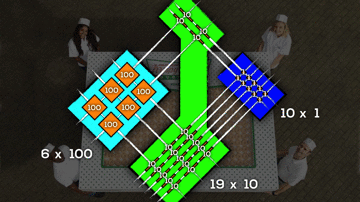#### Multiplication Strategy #5 – Using Japanese Multiplication Without Base Ten Blocks

In this multiplication strategy, we dive into the most common representation of Japanese multiplication where the multiplication is typically completed using sticks or lines without any connection to base ten blocks (where this method is derived from).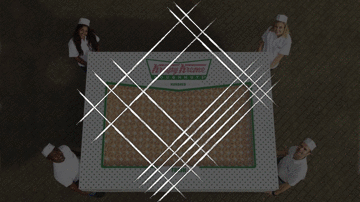#### Multiplication Strategy #6 – Multiplication With Area Models and Partial Products

In this multiplication strategy, we move away from the concrete manipulative completely and work with a visual representation where students represent multiplication as a series of partial products. Students can decompose the factors into friendly “chunks” and then multiply to find the area of each section of the donut box.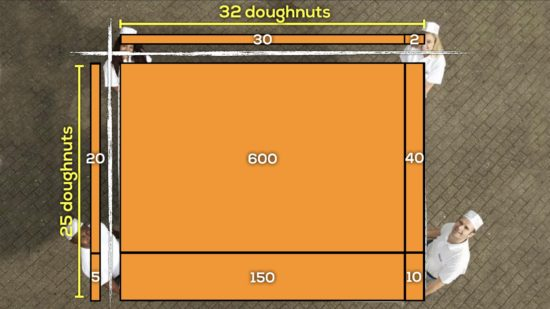#### Multiplication Strategy #7 – Connecting the Area Model to the Standard Algorithm

In this multiplication strategy, we make a jump from a visual representation using an area model to a symbolic representation, the Standard Algorithm as we know it here in Ontario. You’ll quickly notice that base ten blocks, Japanese multiplication and area models all help support the conceptual understanding of the standard algorithm.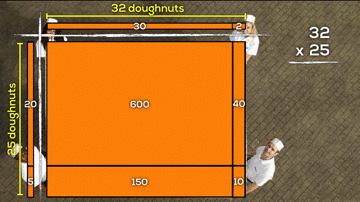Check out my post on the Progression of Multiplication for more tools and representations that can be useful when working with multiplication in your classroom.

### Version 3: Reveal Smaller Box of Donuts Solution

If you used the primary version of this problem, you’ll want to reveal the following so your students can get their moment of glory: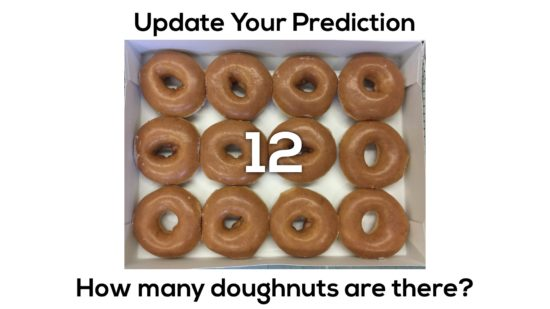## Sequel #1, How Many Layers?

The best part is that the fun has just begun.

If you chose friendly numbers for this task, I’ll show students this image:

If you chose the actual dimensions (less friendly numbers), I’d show students this image: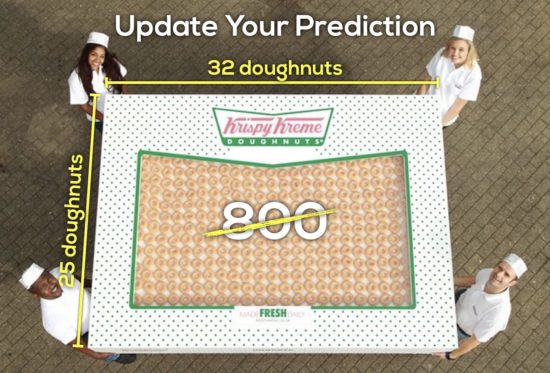Next, I challenge them to determine how many layers of donuts their must be based on what they have done so far. Students already know that one layer is 600 (if friendly numbers were used) or 800 (if the actual dimensions were used), so now they must determine how many layers there are.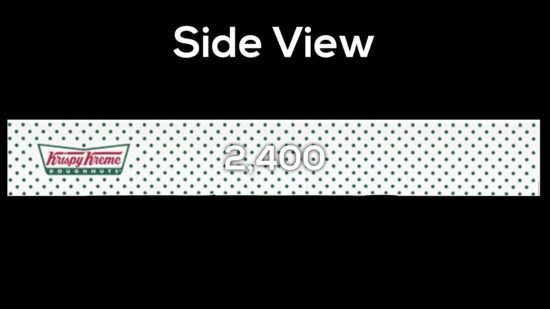So while we know that the double hundred dozen box has 2,400 donuts in it, the number of layers will be different in the friendly number case and in the actual dimensions case.

If students are quite fluent with division and/or the long division algorithm, then the solutions will likely be less than fun to explore. However, if you hit students with this task before introducing long division, it could be a great way to build conceptual understanding using repeated subtraction and open area models:

### Division Strategy: Fair Sharing Groups of 100 Donuts Using Base Ten Flats

In the following division strategy, I’ve attempted to show what students (and teachers) typically resort to when we think about physically dividing objects regardless of whether they are real objects (like donuts) or manipulatives being used to abstractly represent the real object. In the Ontario Grade 2 Math Curriculum, we explicitly use fair sharing as an introduction to division where students are asked to take groups of objects and split them into equal groups. Here’s what that might look like in a classroom where students use the same strategy, but use large units (or groups) for each round of sharing: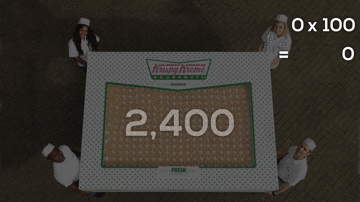### Division Strategy: Repeated Subtraction to Reveal Flexible Division Algorithm

In both versions – using friendly numbers or the actual dimensions – we utilize division as repeated subtraction to help make a connection to a flexible division algorithm which can also be found in our Ontario Guide to Effective Instruction Number Sense and Numeration, Grades 4 to 6: Division resource on page 21. I’ve attempted to make a clearer connection between repeated subtraction using a visual representation and show how it can be connected directly to a symbolic representation that looks mighty close to the long division algorithm.

#### Version #1: Friendly Number Animated Gif:

Here’s the version if you used friendly numbers: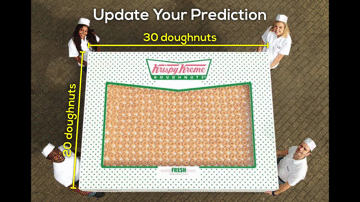Or, consider checking out the friendly number animated gif as a silent solution video here.

#### Version #2: Actual Dimensions Animated Gif:

Here’s the version if you used the actual dimensions: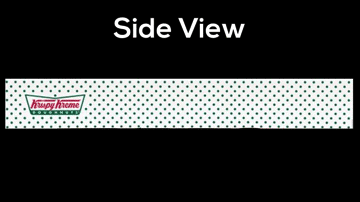## Sequel #2: Fair Share Amongst 8 Classes

If your school bought this box of doughnuts to split between 8 classes, how many would each class get?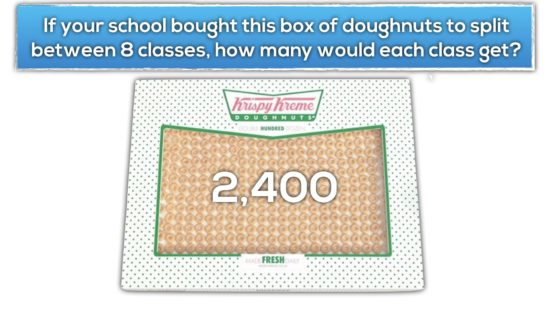If you used friendly numbers for this task, here are some possible strategies that students might consider including using an open area model and flexible division: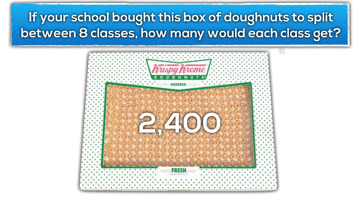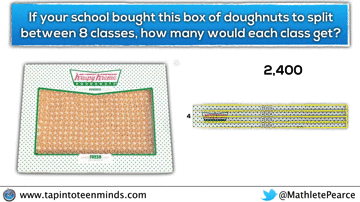If you chose the actual dimensions in order to raise the floor on this task with less friendly numbers, the extension question will result in the same number of donuts for each of the 8 classes. However, some of the representations may look the same, while others may not.

For example, if a student chooses to use repeated subtraction, flexible division or the long division algorithm, both the process and result may look the same.

However, if the student approached the problem by visually dividing each layer into parts, the process may look significantly different than if a student were to use a similar approach in the friendly numbers case.

In the animated gif below, you’ll see an example where a student might attempt dividing each layer into smaller and smaller pieces until there are enough pieces to fair share with all 8 classes.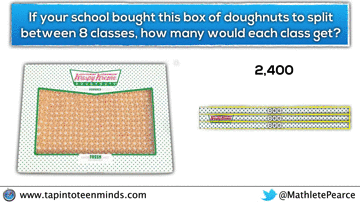## Sequel #3: How Many Calories in the Box?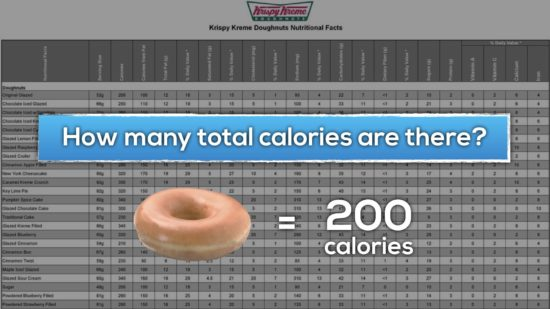## Sequel #4: How Many Calories in the Box?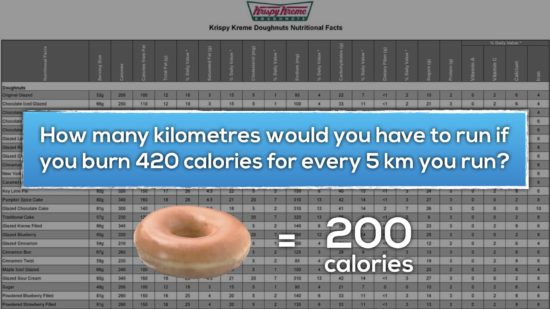## Primary Sequel #5: How Many Donuts Are In 3 Boxes?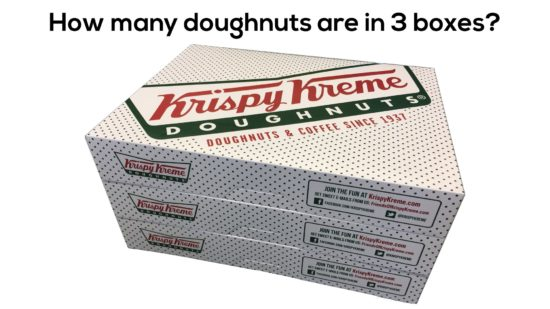Allow them to make some predictions. Possibly show them this image as well: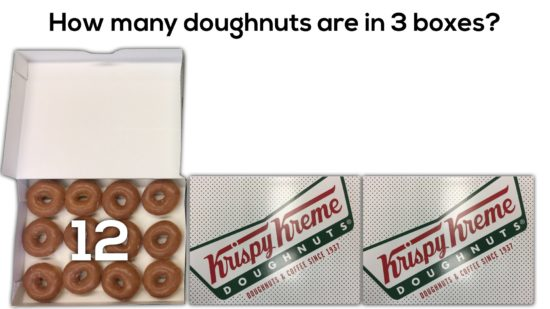After allowing them to use a strategy of their choice, you can reveal the following image: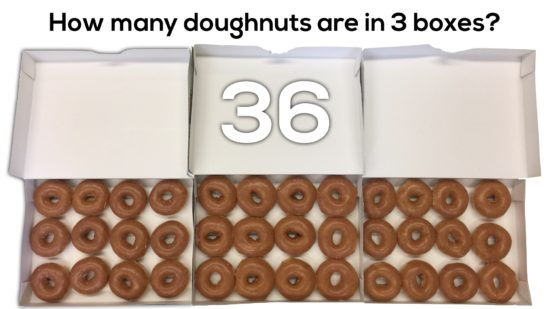## Sequel #6: How Many Donuts In the Texas Flag?

Thanks to Chase Orton for sharing out the following extension image:

I’m immediately wondering some of these:

• How many donuts of each colour?
• What fraction of the flag is red? blue? white?
• What might the ratio / fraction be if you did this with your province / state flag?

The full article, Krispy Kreme Celebrates Texas Independence Day with 1836 Donuts Arranged into the Texas Flag can be found here.

## New to Using 3 Act Math Tasks?

Download the 2-page printable 3 Act Math Tip Sheet to ensure that you have the best start to your journey using 3 Act math Tasks to spark curiosity and fuel sense making in your math classroom!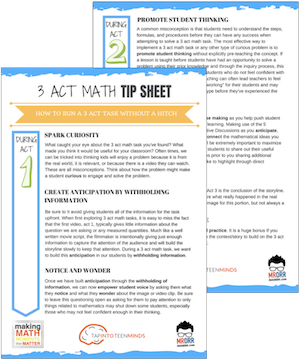## Share With Your Learning Community:I’m Kyle Pearce and I am a former high school math teacher. I’m now the K-12 Mathematics Consultant with the Greater Essex County District School Board, where I uncover creative ways to spark curiosity and fuel sense making in mathematics. Read more.

Access Other Real World Math Tasks

## Search More 3 Act Math Tasks

Spark Curiosity!